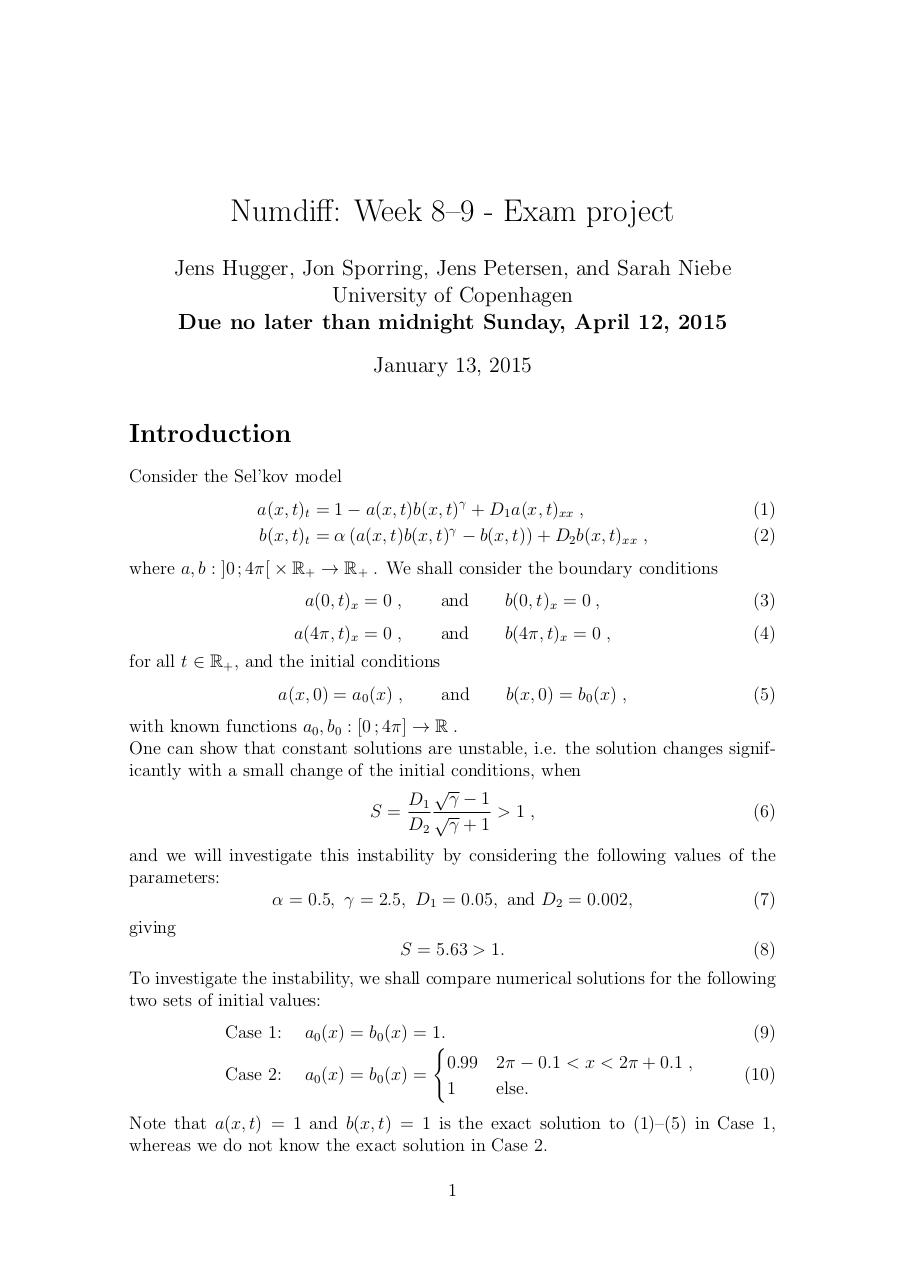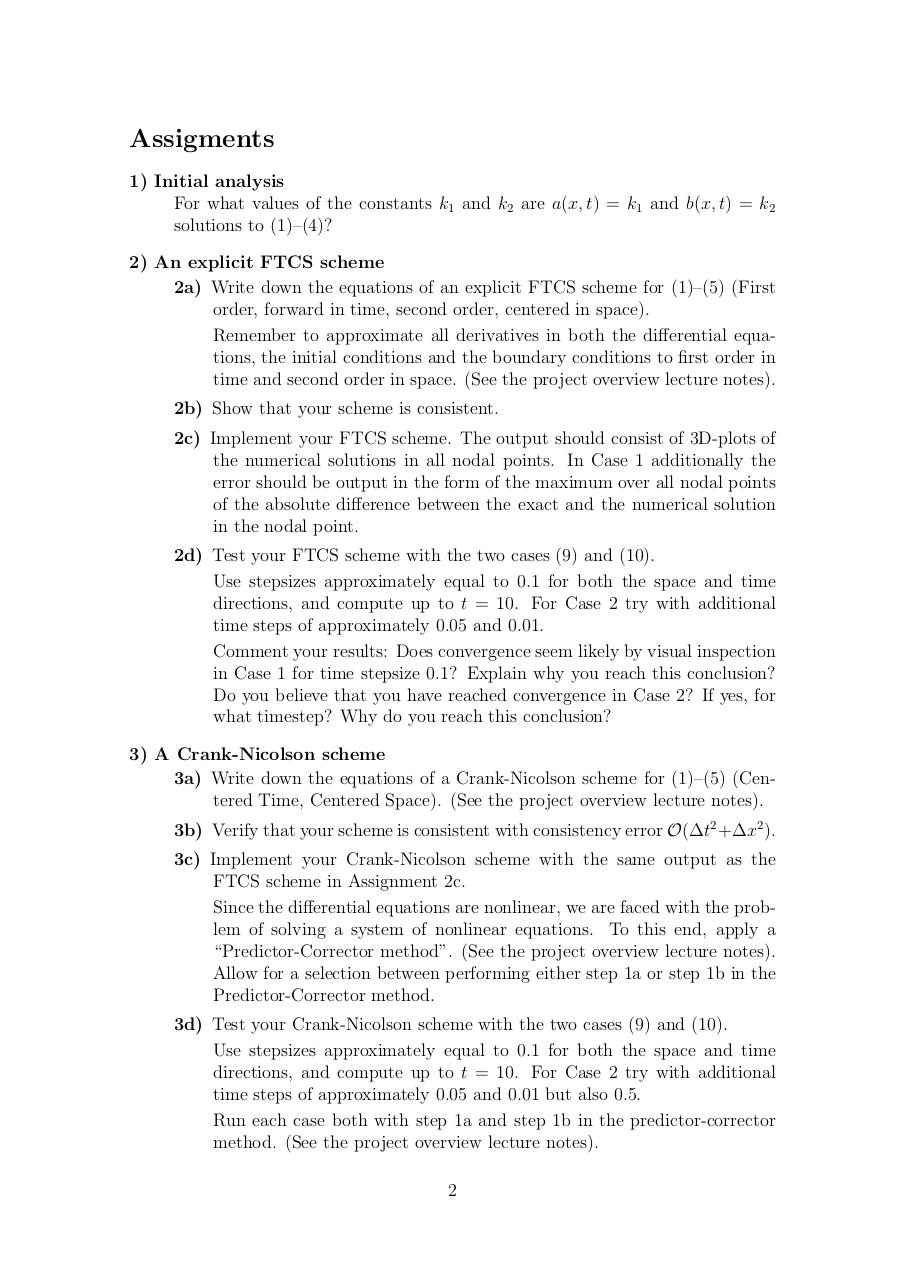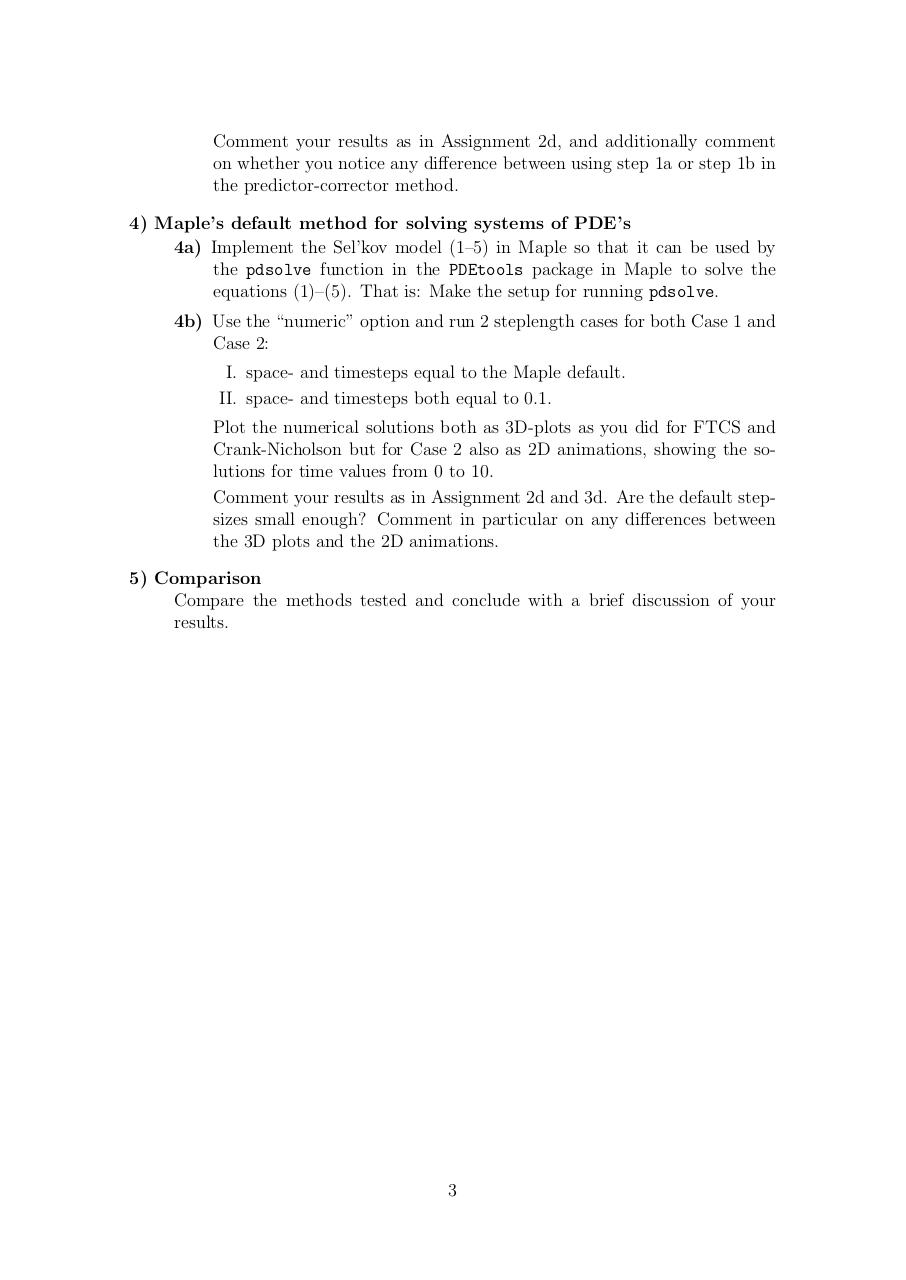# Eksamen .pdf

### File information

Original filename: Eksamen.pdf

This PDF 1.5 document has been generated by TeX / MiKTeX pdfTeX-1.40.14, and has been sent on pdf-archive.com on 07/03/2016 at 13:04, from IP address 80.71.x.x. The current document download page has been viewed 440 times.
File size: 106 KB (3 pages).
Privacy: public file

Eksamen.pdf (PDF, 106 KB)

### Document preview

Numdiff: Week 8–9 - Exam project
Jens Hugger, Jon Sporring, Jens Petersen, and Sarah Niebe
University of Copenhagen
Due no later than midnight Sunday, April 12, 2015
January 13, 2015

Introduction
Consider the Sel’kov model
a(x, t)t = 1 − a(x, t)b(x, t)γ + D1 a(x, t)xx ,
b(x, t)t = α (a(x, t)b(x, t)γ − b(x, t)) + D2 b(x, t)xx ,

(1)
(2)

where a, b : ]0 ; 4π[ × R+ → R+ . We shall consider the boundary conditions
a(0, t)x = 0 ,

and

b(0, t)x = 0 ,

(3)

a(4π, t)x = 0 ,

and

b(4π, t)x = 0 ,

(4)

and

b(x, 0) = b0 (x) ,

(5)

for all t ∈ R+ , and the initial conditions
a(x, 0) = a0 (x) ,

with known functions a0 , b0 : [0 ; 4π] → R .
One can show that constant solutions are unstable, i.e. the solution changes significantly with a small change of the initial conditions, when

D1 γ − 1
&gt;1,
(6)
S=

D2 γ + 1
and we will investigate this instability by considering the following values of the
parameters:
α = 0.5, γ = 2.5, D1 = 0.05, and D2 = 0.002,
(7)
giving
S = 5.63 &gt; 1.

(8)

To investigate the instability, we shall compare numerical solutions for the following
two sets of initial values:
Case 1:
Case 2:

a0 (x) = b0 (x) = 1.
(
0.99 2π − 0.1 &lt; x &lt; 2π + 0.1 ,
a0 (x) = b0 (x) =
1
else.

(9)
(10)

Note that a(x, t) = 1 and b(x, t) = 1 is the exact solution to (1)–(5) in Case 1,
whereas we do not know the exact solution in Case 2.
1

Assigments
1) Initial analysis
For what values of the constants k1 and k2 are a(x, t) = k1 and b(x, t) = k2
solutions to (1)–(4)?
2) An explicit FTCS scheme
2a) Write down the equations of an explicit FTCS scheme for (1)–(5) (First
order, forward in time, second order, centered in space).
Remember to approximate all derivatives in both the differential equations, the initial conditions and the boundary conditions to first order in
time and second order in space. (See the project overview lecture notes).
2b) Show that your scheme is consistent.
2c) Implement your FTCS scheme. The output should consist of 3D-plots of
the numerical solutions in all nodal points. In Case 1 additionally the
error should be output in the form of the maximum over all nodal points
of the absolute difference between the exact and the numerical solution
in the nodal point.
2d) Test your FTCS scheme with the two cases (9) and (10).
Use stepsizes approximately equal to 0.1 for both the space and time
directions, and compute up to t = 10. For Case 2 try with additional
time steps of approximately 0.05 and 0.01.
Comment your results: Does convergence seem likely by visual inspection
in Case 1 for time stepsize 0.1? Explain why you reach this conclusion?
Do you believe that you have reached convergence in Case 2? If yes, for
what timestep? Why do you reach this conclusion?
3) A Crank-Nicolson scheme
3a) Write down the equations of a Crank-Nicolson scheme for (1)–(5) (Centered Time, Centered Space). (See the project overview lecture notes).
3b) Verify that your scheme is consistent with consistency error O(∆t2 +∆x2 ).
3c) Implement your Crank-Nicolson scheme with the same output as the
FTCS scheme in Assignment 2c.
Since the differential equations are nonlinear, we are faced with the problem of solving a system of nonlinear equations. To this end, apply a
“Predictor-Corrector method”. (See the project overview lecture notes).
Allow for a selection between performing either step 1a or step 1b in the
Predictor-Corrector method.
3d) Test your Crank-Nicolson scheme with the two cases (9) and (10).
Use stepsizes approximately equal to 0.1 for both the space and time
directions, and compute up to t = 10. For Case 2 try with additional
time steps of approximately 0.05 and 0.01 but also 0.5.
Run each case both with step 1a and step 1b in the predictor-corrector
method. (See the project overview lecture notes).
2

on whether you notice any difference between using step 1a or step 1b in
the predictor-corrector method.
4) Maple’s default method for solving systems of PDE’s
4a) Implement the Sel’kov model (1–5) in Maple so that it can be used by
the pdsolve function in the PDEtools package in Maple to solve the
equations (1)–(5). That is: Make the setup for running pdsolve.
4b) Use the “numeric” option and run 2 steplength cases for both Case 1 and
Case 2:
I. space- and timesteps equal to the Maple default.
II. space- and timesteps both equal to 0.1.
Plot the numerical solutions both as 3D-plots as you did for FTCS and
Crank-Nicholson but for Case 2 also as 2D animations, showing the solutions for time values from 0 to 10.
Comment your results as in Assignment 2d and 3d. Are the default stepsizes small enough? Comment in particular on any differences between
the 3D plots and the 2D animations.
5) Comparison
Compare the methods tested and conclude with a brief discussion of your
results.

3#### HTML Code

Copy the following HTML code to share your document on a Website or Blog

#### QR Code### Related keywords